### 9.5.1.1 Comparing rewards

Imagine assigning reward values to various outcomes of a decision-making process. In some applications numerical values may come naturally. For example, the reward might be the amount of money earned in a financial investment. In robotics applications, one could negate time to execute a task or the amount of energy consumed. For example, the reward could indicate the amount of remaining battery life after a mobile robot builds a map.

In some applications the source of rewards may be subjective. For example, what is the reward for washing dishes, in comparison to sweeping the floor? Each person would probably assign different rewards, which may even vary from day to day. It may be based on their enjoyment or misery in performing the task, the amount of time each task would take, the perceptions of others, and so on. If decision theory is used to automate the decision process for a human client,'' then it is best to consult carefully with the client to make sure you know their preferences. In this situation, it may be possible to sort their preferences and then assign rewards that are consistent with the ordering.

Once the rewards are assigned, consider making a decision under Formulation 9.1, which does not involve nature. Each outcome corresponds directly to an action,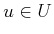. If the rewards are given by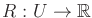, then the cost,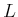, can be defined as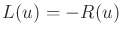for every. Satisfying the client is then a matter of choosingto minimize.

Now consider a game against nature. The decision now involves comparing probability distributions over the outcomes. The space of all probability distributions may be enormous, but this is simplified by using expectation to map each probability distribution (or density) to a real value. The concern should be whether this projection of distributions onto real numbers will fail to reflect the true preferences of the client. The following example illustrates the effect of this.

Example 9..22 (Do You Like to Gamble?)   Suppose you are given three choices:
1. You can have 1000 Euros.
2. We will toss an unbiased coin, and if the result is heads, then you will receive 2000 Euros. Otherwise, you receive nothing.
3. With probability 2/3, you can have 3000 Euros; however, with probability 1/3, you have to give me 3000 Euros.
The expected reward for each of these choices is 1000 Euros, but would you really consider these to be equivalent? Your love or disdain for gambling is not being taken into account by the expectation. How should such an issue be considered in games against nature?To begin to fix this problem, it is helpful to consider another scenario. Many people would probably agree that having more money is preferable (if having too much worries you, then you can always give away the surplus to your favorite charities). What is interesting, however, is that being wealthy decreases the perceived value of money. This is illustrated in the next example.

Example 9..23 (Reality Television)   Suppose you are lucky enough to appear on a popular reality television program. The point of the show is to test how far you will go in making a fool out of yourself, or perhaps even torturing yourself, to earn some money. You are asked to do some unpleasant task (such as eating cockroaches, or holding your head under water for a long time, and so on.). Let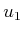be the action to agree to do the task, and let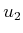mean that you decline the opportunity. The prizes are expressed in U.S. dollars. Imagine that you are a starving student on a tight budget.

Below are several possible scenarios that could be presented on the television program. Consider how you would react to each one.

1. Suppose thatearns you $1 andearns you nothing. Purely optimizing the reward would lead to choosing, which means performing the unpleasant task. However, is this worth$1? The problem so far is that we are not taking into account the amount of discomfort in completing a task. Perhaps it might make sense to make a reward function that shifts the dollar values by subtracting the amount for which you would be just barely willing to perform the task.

2. Suppose thatearns you $10,000 andearns you nothing.$10,000 is assumed to be an enormous amount of money, clearly worth enduring any torture inflicted by the television program. Thus,is preferable.

3. Now imagine that the television host first gives you $10 million just for appearing on the program. Are you still willing to perform the unpleasant task for an extra$10,000? Probably not. What is happening here? Your sense of value assigned to money seems to decrease as you get more of it, right? It would not be too interesting to watch the program if the contestants were all wealthy oil executives.

4. Suppose that you have performed the task and are about to win the prize. Just to add to the drama, the host offers you a gambling opportunity. You can select actionand receive $10,000, or be a gambler by selectingand have probability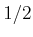of winning$25,000 by the tossing of a fair coin. In terms of the expected reward, the clear choice is. However, you just completed the unpleasant task and expect to earn money. The risk of losing it all may be intolerable. Different people will have different preferences in this situation.

5. Now suppose once again that you performed the task. This time your choices are, to receive $100, or, to have probabilityof receiving$250 by tossing a fair coin. The host is kind enough, though, to let you play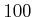times. In this case, the expected totals for the two actions are $10,000 and$12,500, respectively. This time it seems clear that the best choice is to gamble. Afterindependent trials, we would expect that, with extremely high probability, over \$10,000 would be earned. Thus, reasoning by expected-case analysis seems valid if we are allowed numerous, independent trials. In this case, with high probability a value close to the expected reward should be received.Based on these examples, it seems that the client or evaluator of the decision-making system must indicate preferences between probability distributions over outcomes. There is a formal way to ensure that once these preferences are assigned, a cost function can be designed for which its expectation faithfully reflects the preferences over distributions. This results in utility theory, which involves the following steps:

1. Require that the client is rational when assigning preferences. This notion is defined through axioms.
2. If the preferences are assigned in a way that is consistent with the axioms, then a utility function is guaranteed to exist. When expected utility is optimized, the preferences match exactly those of the client.
3. The cost function can be derived from the utility function.

The client must specify preferences among probability distributions of outcomes. Suppose that Formulation 9.2 is used. For convenience, assume that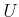and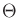are finite. Letdenote a state space based on outcomes.9.5 Let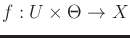denote a mapping that assigns a state to every outcome. A simple example is to declare that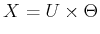and make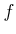the identity map. This makes the outcome space and state space coincide. It may be convenient, though, to useto collapse the space of outcomes down to a smaller set. If two outcomes map to the same state using, then it means that the outcomes are indistinguishable as far as rewards or costs are concerned.

Let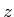denote a probability distribution over, and let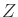denote the set of all probability distributions over. Every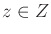is represented as an-dimensional vector of probabilities in which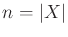; hence, it is considered as an element of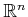. This makes it convenient to blend'' two probability distributions. For example, let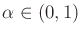be a constant, and let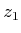and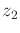be any two probability distributions. Using scalar multiplication, a new probability distribution,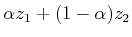, is obtained, which is a blend ofand. Conveniently, there is no need to normalize the result. It is assumed thatandinitially have unit magnitude. The blend has magnitude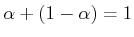.

The modeler of the decision process must consult the client to represent preferences among elements of. Let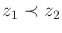mean thatis strictly preferred over. Let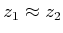mean thatandare equivalent in preference. Letmean that eitheror. The following example illustrates the assignment of preferences.

Example 9..24 (Indicating Preferences)   Suppose that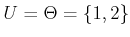, which leads to four possible outcomes: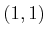,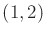,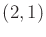, and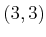. Imagine that nature represents a machine that generatesor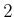according to a probability distribution. The action is to guess the number that will be generated by the machine. If you pick the same number, then you win that number of gold pieces. If you do not pick the same number, then you win nothing, but also lose nothing.

Consider the construction of the state spaceby using. The outcomesandare identical concerning any conceivable reward. Therefore, these should map to the same state. The other two outcomes are distinct. The state space therefore needs only three elements and can be defined as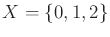. Let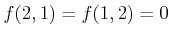,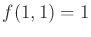, and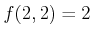. Thus, the last two states indicate that some gold will be earned.

The setof probability distributions overis now considered. Eachis a three-dimensional vector. As an example,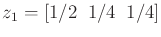indicates that the state will be 0 with probability,with probability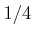, andwith probability. Suppose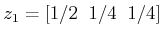. Which distribution would you prefer? It seems in this case thatis uniformly better thanbecause there is a greater chance of winning gold. Thus, we declare. The distribution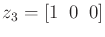seems to be the worst imaginable. Hence, we can safely declare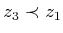and.

The procedure of determining the preferences can become quite tedious for complicated problems. In the current example,is a 2D subset of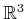. This subset can be partitioned into a finite set of regions over which the client may be able to clearly indicate preferences. One of the major criticisms of this framework is the impracticality of determining preferences over.

After the preferences are determined, is there a way to ensure that a real-value function onexists for which the expected value exactly reflects the preferences? If the axioms of rationality are satisfied by the assignment of preferences, then the answer is yes. These axioms are covered next.Steven M LaValle 2020-08-14